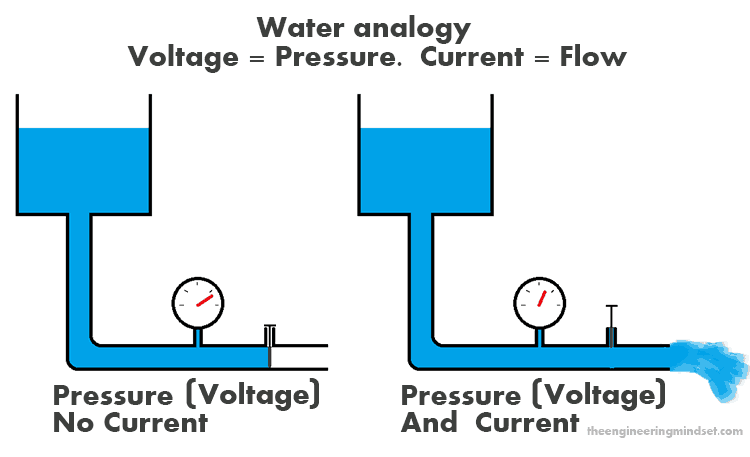# What is Voltage?

Potential difference or voltage, Explained.

4
14607Voltage is the driving force of current, it is the electromotive force which pushes free electrons from one atom to another in the same direction. Confused by this? if so then click here to first learn how electricity works.

### How to imagine Voltage

It is easiest to think of voltage like pressure in a pipe. When there is a higher voltage (pressure) then a stronger current will flow. Although it is important to understand that voltage (pressure) can exist without a current (flow), but current cannot exist without voltage (pressure).Voltage is often referred to as potential difference, this is because there will be a difference in the potential energy of the electrons, between any two points in a circuit. As electrons flow through a battery, their potential energy is increased but as they flow through a light bulb, their potential energy will decrease, this energy will leave the circuit as light and heat.

Take a regular 1.5V AA Battery for example, between it’s two terminals (+ and -) there’s a potential difference of 1.5 Volts.Voltage, or potential difference, is simply a measurement of the amount of energy (Joules) per unit of charge (Coulomb). E.g. in a 1.5V AA battery, every coulomb (charge) will receive 1.5 volts, or Joules of energy.

Voltage = Joules ÷ Coulomb

1 Volt = 1 Joule per coulomb

100 Volts = 100 Joules per coulomb

1 coulomb = 6,200,000,000,000,000,000 electrons (6.2×1018)

#### Measuring Voltage

We measure voltage in the unit of “Volts” which will usually be represented simply by the letter “V” on drawings and technical literature. It is often necessary to quantify an amount of voltage, this is done so according to SI units, the most common quantities of voltage you will see are:

• megavolts (MV)
• kilovolts (kV)
• volts (V)
• millivolts (mV)
• microvolts (μV)

Voltage is always measured across two points with a device called a Voltmeter. Voltmeters are either analog of digital, the latter being the most accurate. Voltmeters are usually built into portable digital multimeter devices, as illustrated below, they are a common and often essential tool for any electrician or electrical engineer. You will usually find analog voltmeters on older electrical panels such as switchboards and generators, but almost all new equipment will come with digital meters as standard.Portable digital multimeter with a voltmeter functionAnalog Voltmeter

On electrical schematics, you will see voltmeter devices denoted by the letter V within a circle, as illustrated below:Voltmeter symbol

#### Calculating Voltage

In electrical circuits, the voltage can be calculated according to Ohm’s triangle. To find voltage (V) simply multiply current (I) by resistance (R).

Voltage = Current / Resistance

## Example

Current in circuit  = 10Amps
Resistance of circuit =

Voltage = 10A x

Answer: 26V

.## Summary

• Voltage is the force which moves electrons from one atom to another
• Voltage is also known as potential difference
• Voltage is measured by the unit “volts” (V)
• Batteries increase potential energy of electrons
• Light bulbs, and other loads, decrease electron potential energy
• Voltage is measured with a voltmeter
• The Voltage of a circuit can be calculated by multiplying the current and resistance

#### 4 COMMENTS

1.John

Typo: under “calculating voltage” you have the equation as Voltage = Current / Resistance when it’s really Voltage = Current * Resistance.

2. I displayed the data given in this article for teaching high school kids. I showed them the pictures which made them understand the concept easily. They liked the article posted by the author. They didn’t feel bored unlike reading the textbooks.

3.Michele

Under “calculating voltage” your example shows that 10A * 2 ohm’s = 26
– I’m confused, 10(2) = 20. How did you get 26?

• Looks like you’ve spotted a mistake. I’ll correct it now, thank you for letting me know.